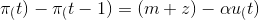### Create an Account

Already have account?

### Forgot Your Password ?

Home / Questions / If the Phillips curve equation is represented by equation : \pi _(t)-\pi _(t-1)=(m+z)-\alp...

# If the Phillips curve equation is represented by equation : \pi _(t)-\pi _(t-1)=(m+z)-\alpha u_(t) , with the change in the inflation rate equal to m plus z minus the product of alpha and u, the natur

If the Phillips curve equation is represented by equation :, with the change in the inflation rate equal to m plus z minus the product of alpha and u, the natural rate of unemployment will be equal to...

a. m + z.

b. m + z - alpha.

c. 0.

d. none of the above

Apr 16 2021 View more View Less

#### Answer (Solved)Subscribe To Get Solution